# Force from a Jet of Water A jet of fluid striking a vane exerts a force on the vane it is equal a...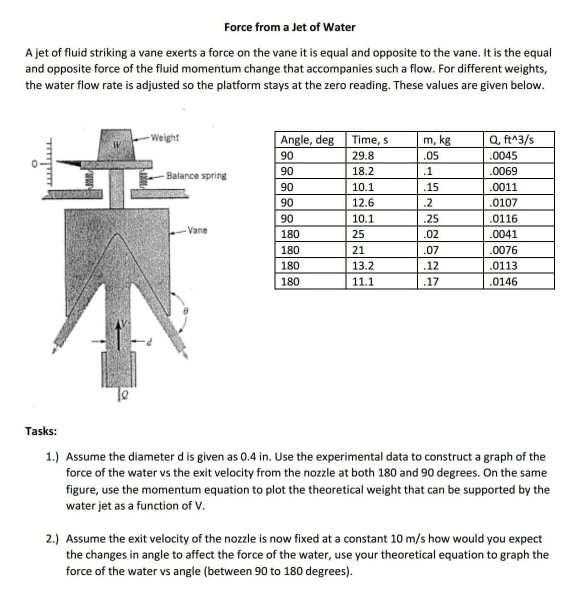Force from a Jet of Water A jet of fluid striking a vane exerts a force on the vane it is equal and opposite to the vane. It is the equal and opposite force of the fluid momentum change that accompanies such a flow. For different weights, the water flow rate is adjusted so the platform stays at the zero reading. These values are given below Q, ftA3/s 0045 0069 0011 0107 0116 0041 0076 0113 0146 Weight Angle, deg 90 Time, s 29.8 18.2 05 Balance spring 15 90 90 180 180 180 180 12.6 10.1 25 21 13.2 11.1 25 02 07 12 17 Vane Tasks: 1) Assume the diameter d is given as 0.4 in. Use the experimental data to construct a graph of the force of the water vs the exit velocity from the nozzle at both 180 and 90 degrees. On the same figure, use the momentum equation to plot the theoretical weight that can be supported by the water jet as a function of V 2.) Assume the exit velocity of the nozzle is now fixed at a constant 10 m/s how would you expect the changes in angle to affect the force of the water, use your theoretical equation to graph the force of the water vs angle (between 90 to 180 degrees)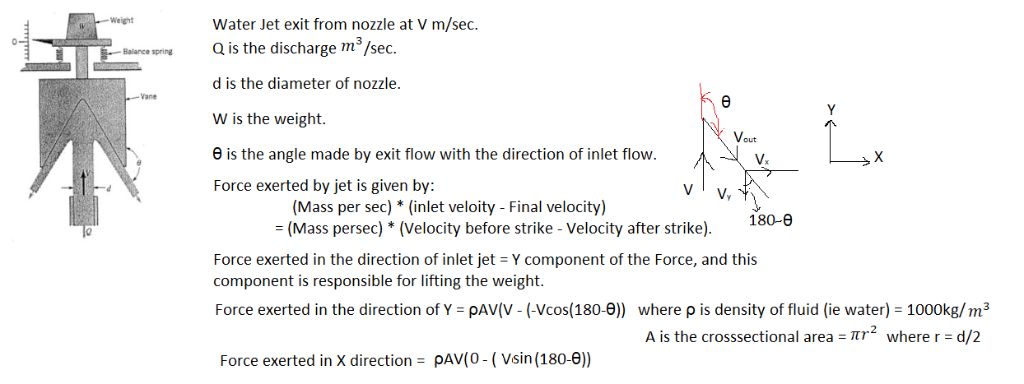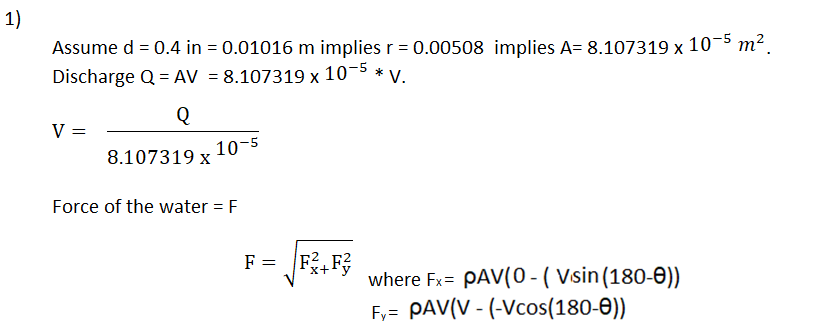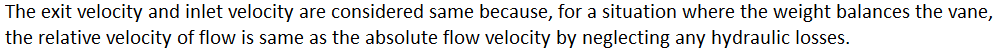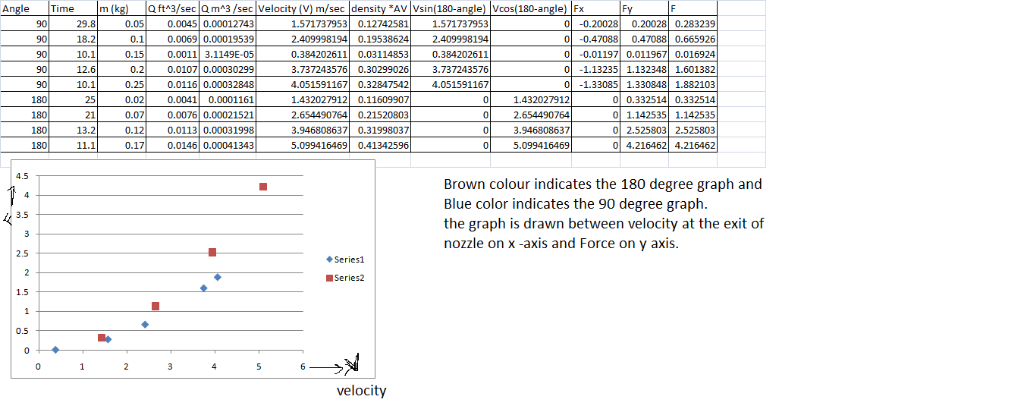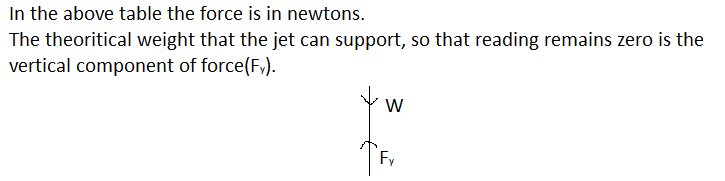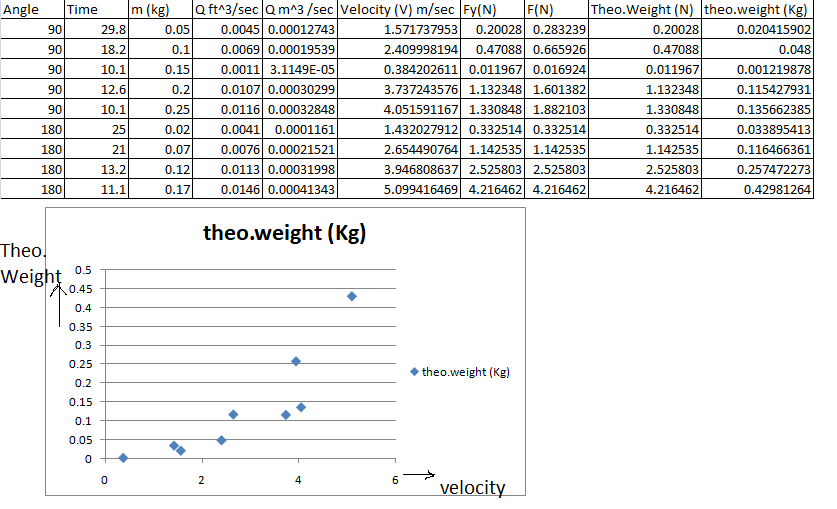2)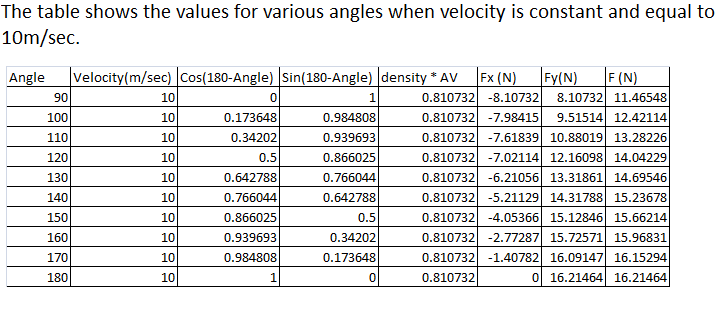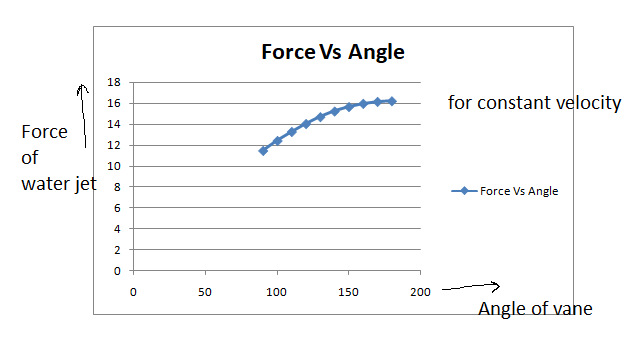##### Add Answer of: Force from a Jet of Water A jet of fluid striking a vane exerts a force on the vane it is equal a...
More Homework Help Questions Additional questions in this topic.